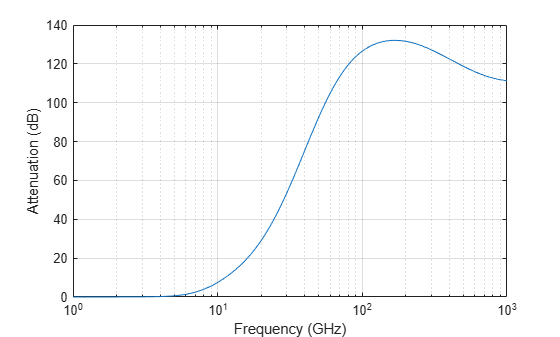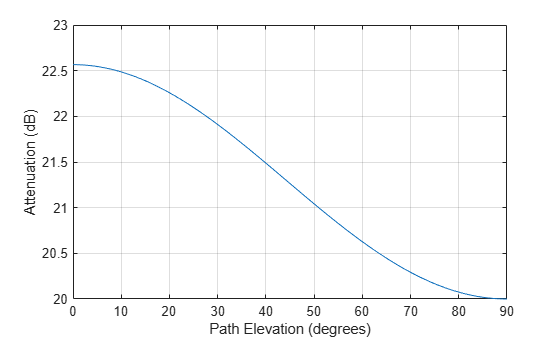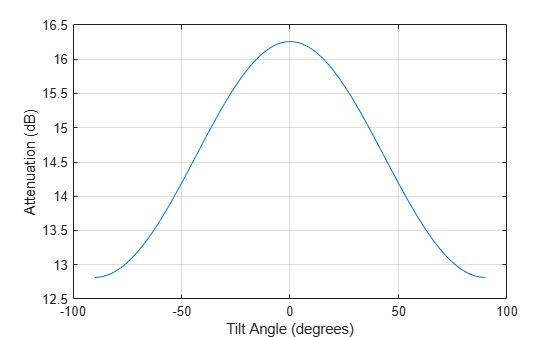# cranerainpl

RF signal attenuation due to rainfall using Crane model

## Syntax

``L = cranerainpl(range,freq,rainrate)``
``L = cranerainpl(range,freq,rainrate,elev)``
``L = cranerainpl(range,freq,rainrate,elev,tau)``

## Description

example

````L = cranerainpl(range,freq,rainrate)` returns the signal attenuation, `L`, due to rain based on the Crane rain model . Signal attenuation is a function of the signal path length, `range`, the signal frequency, `freq`, and the rain rate, `rainrate`. The rain rate is defined as the long-term statistical rain rate. The attenuation model applies only for frequencies from 1 GHz to 1000 GHz and is valid for ranges up to 22.5 km. The Crane model accounts for the cellular nature of rainstorms.```

example

````L = cranerainpl(range,freq,rainrate,elev)` also specifies the elevation angle, `elev`, of the signal path.```

example

````L = cranerainpl(range,freq,rainrate,elev,tau)` also specifies the polarization tilt angle, `tau`, of the signal.```

## Examples

collapse all

Use the Crane rain model to compute the signal attenuation caused by rain for a 20 GHz signal sent over a distance of 10 km. Use rain rates of 10.0 and 100.0 mm/hr.

First, set the rain rate to 10 mm/hr.

```rr = 10.0; L = cranerainpl(10e3,20.0e9,rr)```
```L = 12.5988 ```

Repeat the computation using a rain rate of 100.0 mm/hr.

```rr = 100.0; L = cranerainpl(10e3,20.0e9,rr)```
```L = 73.1912 ```

Plot the signal attenuation due to rain for signals in the frequency range from 1 to 1000 GHz. Use the Crane model to compute the attenuation for a rain rate of 30.0 mm/hr and a signal path distance of 10 km.

```rr = 30.0; freq = [1:1000]*1e9; L = cranerainpl(10e3,freq,rr); semilogx(freq/1e9,L) grid xlabel('Frequency (GHz)') ylabel('Attenuation (dB)')```Plot the signal attenuation due to rain as a function of elevation angle. Elevation angles vary from 0 to 90 degrees. Assume a path distance of 10 km and a signal frequency of 10 GHz. The rain rate is 100 mm/hr.

`rr = 100.0;`

Set the elevation angles, frequency, and path length.

```elev = [0:1:90]; freq = 10.0e9; rng = 10e3*ones(size(elev));```

Compute and plot the loss.

```L = cranerainpl(rng,freq,rr,elev); plot(elev,L) grid xlabel('Path Elevation (degrees)') ylabel('Attenuation (dB)')```Plot the signal attenuation due to rainfall as a function of the polarization tilt angle. Assume a path distance of 10 km, a signal frequency of 10 GHz, and a path elevation angle of 0 degrees. Set the rainfall rate to 70 mm/hour. Plot the signal attenuation against polarization tilt angle.

Set the polarization tilt angle to vary from -90 to 90 degrees.

`tau = -90:90;`

Set the elevation angle, frequency, path distance, and rain rate.

```elev = 0; freq = 10.0e9; rng = 10e3*ones(size(tau)); rr = 70.0;```

Compute and plot the attenuation.

```L = cranerainpl(rng,freq,rr,elev,tau); plot(tau,L) grid xlabel('Tilt Angle (degrees)') ylabel('Attenuation (dB)')```## Input Arguments

collapse all

Signal path length, specified as a positive scalar, a real-valued 1-by-M vector of positive values, or real-valued M-by-1 vector of positive values. Units are in meters.

Example: `[13000.0,14000.0]`

Signal frequency, specified as a positive scalar, a real-valued 1-by-N vector of positive values, or a real-valued N-by-1 vector of positive values. Units are in Hz. Frequencies must lie in the range 1–1000 GHz.

Example: `[2.0:2:10.0]*1e9`]

Rain rate, specified as a nonnegative scalar. Rain rate represents the long-term statistical rainfall rate provided by Crane (see ). Units are in mm/hr.

Example: `100.5`

Signal path elevation angle, specified as a real-valued scalar, or real-valued M-by-1 or real-valued 1-by-M vector. Units are in degrees between –90° and 90°.

• If `elev` is a scalar, all propagation paths have the same elevation angle.

• If `elev` is a vector, its length must match the length of `range` and each element in `elev` corresponds to a propagation range.

Example: `[0,45]`

Tilt angle of the signal polarization ellipse, specified as a scalar, a real-valued 1-by-M vector, or a real-valued M-by-1 vector. Tilt angle values are in the range –90° and 90°, inclusive. Units are in degrees.

• If `tau` is a scalar, all signals have the same tilt angle.

• If `tau` is a vector, its length must match the length of `range`. In that case, each element in `tau` corresponds to a propagation path in `range`.

The tilt angle is defined as the angle between the semimajor axis of the polarization ellipse and the x-axis. Because the ellipse is symmetrical, a tilt angle of 10° corresponds to the same polarization state as a tilt angle of -80°. Thus, the tilt angle need only be specified between ±90°.

Example: `[45,30]`

## Output Arguments

collapse all

Signal attenuation, returned as a real-valued M-by-N matrix. Each matrix row represents a different path where M is the number of paths. Each column represents a different frequency where N is the number of frequencies. Units are in dB.

collapse all

### Crane Rainfall Attenuation Model

The Crane model calculates the attenuation of signals that propagate through regions of rainfall. The model was developed for use on Earth–space or terrestrial propagation paths and is a commonly-used method for the calculation of rain attenuation. The model is based on observations of rain rate, rain structure, and the vertical variation of temperature in the atmosphere. The Crane model (see Electromagnetic Wave Propagation through Rain) is primarily applicable to North America. The Crane model generally predicts losses greater than those of the ITU rain attenuation model used in the `rainpl` function. However, the uncertainty of both models and the short-term variation of fade can be large.

The ITU and Crane models are very similar but have some differences. The ITU and Crane rain attenuation models both require statistical annual rainfall rates and utilize an effective path length reduction factor to account for the cellular nature of storms. The 0.01% rainfall rate tables provided by Crane and the ITU are different. The Crane rainfall zones are similar to the ITU zones but more zones are defined in the US than in the ITU model. The ITU rainfall zones are discussed in ITU-R P.838-3: Specific attenuation model for rain for use in prediction methods. The Crane model is more complex consisting of a piecewise combination of path profiles composed of exponential functions.

The Crane model utilizes two exponential functions to span the distance from 0 to 22.5 km.

• For δ < D < 22.5,

`$L=\gamma \left(\frac{{e}^{y\delta }-1}{y}-\frac{{b}^{\alpha }{e}^{z\delta }}{z}+\frac{{b}^{\alpha }{e}^{zD}}{z}\right)$`

• For 0 < D < δ,

`$L=\gamma \left(\frac{{e}^{yD}-1}{y}\right)$`

where

• L = path attenuation (dB)

• 𝐷 = propagation distance (km)

• R = statistical 0.01% rain rate (mm/hr)

• γ = specific attenuation identical to that calculated in `rainpl`.

`${\gamma }_{R}=k{R}^{\alpha },$`

The parameters k and α depend on the frequency, the polarization state, and the elevation angle of the signal path. These coefficients, given by both Crane Electromagnetic Wave Propagation through Rain and the ITU-R P.838-3: Specific attenuation model for rain for use in prediction methods, are identical and are valid from 1 GHz to 1000 GHz. The specific attenuation model is valid for frequencies from 1–1000 GHz. Rainfall specific attenuation is computed according to the ITU rainfall model in ITU-R P.838-3: Specific attenuation model for rain for use in prediction methods.

The remaining parameters are empirical constants defined as:

• b = 2.3R-0.17

• c = 0.026 - 0.03ln R

• δ = 3.8 - 0.6 ln R

• u = ln (be)/δ

• y = αu

• z = αc

To compute the total attenuation for narrowband signals along a path, the function multiplies the specific attenuation by the propagation distance.

You can also apply the attenuation model to wideband signals. First, divide the wideband signal into frequency subbands and apply attenuation to each subband. Then, sum all attenuated subband signals into the total attenuated signal.

 Crane, Robert K. Electromagnetic Wave Propagation through Rain. Wiley, 1996.

 Radiocommunication Sector of International Telecommunication Union. Recommendation ITU-R P.838-3: Specific attenuation model for rain for use in prediction methods. P Series, Radiowave Propagation 2005.

 Radiocommunication Sector of International Telecommunication Union. Recommendation ITU-R P.530-17: Propagation data and prediction methods required for the design of terrestrial line-of-sight systems. 2017.

 Radiocommunication Sector of International Telecommunication Union. Recommendation ITU-R P.837-7: Characteristics of precipitation for propagation modelling. 6/2017